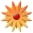# Stations: Equation of a line and Slope

Page Views: 590

Email This Lesson Plan to Me
Overall Rating:(5.0 stars, 1 ratings)

 Keywords: Math Subject(s): Math Grade 8 NETS-S Standard: Critical Thinking, Problem Solving, and Decision MakingView Full Text of Standards School: Franklinton Junior High School, Franklinton, LA Planned By: Amber Painter Original Author: Amber Painter, Franklinton
***Throughout the lesson students may receive or have points deducted on their Classcraft account (an interactive classroom management app).
Standards: Louisiana Student Standards 8th grade Math
B. Understand the connections between proportional relationships, lines, and linear equations. 5. Graph proportional relationships, interpreting the unit rate as the slope of the graph. Compare two different proportional relationships represented in different ways. For example, compare a distance-time graph to a distance-time equation to determine which of two moving objects has greater speed.
6. Use similar triangles to explain why the slope m is the same between any two distinct points on a non-vertical line in the coordinate plane; derive the equation y = mx for a line through the origin and the equation y = mx + b for a line intercepting the vertical axis at b.

Goal #1: The student will be able to distinguish between positive, negative, zero and undefined slopes. The student will be able to manipulate a line to change its slope and y�intercept.
Station 1: In this station students will work with a partner to manipulate lines. The student will work on tablets using the app SLOPE SLIDER. Using the graph on the app, the students will alter the slant and location of the line using dials. The student will shift the line to create a line with a positive slope, a negative slope, slope of zero and an undefined slope. The students will take a picture of each graph and write the equation of the line on their handout. The students will then have to shift the line to change the y�intercept to �4 and again to 3. They will take a picture and record the equation of the lines on their handout.
Goal #2: The student will be able to calculate the slope of a line from two ordered pairs.
Station 2: The student will work with a partner to determine the slope from two points on their handout.
Students must show all steps. Once the students have calculated the slope for all six sets of ordered pairs, they will use the app POLATTA to check their work. In this app, they will enter the two ordered pairs, and the equation of the line will come on the screen. Students will check their answers. If any are incorrect, they must rework them showing all steps and circling their mistake. This allows them to determine their mistakes on their own.
Goal #3: The student will be able to match graphs to their linear equations and determine slope from two points.
Station 3: The student will work independently to show their understanding of graphs, and their linear equations as well as slope. TSW will use tablets to complete a Kahoot! activity matching linear equations to their correct graph and determining slope from a graph and two ordered pairs. Each question is timed, and the students will see the correct answer after the time is up. Once they have completed all ten questions, the students will see their results. Each student must write a statement explaining their score and what they feel they still need help with. The teacher will also have all data from KAHOOT! to see where students are struggling.

Goal #4: The student will be able to graph lines in the form y=mx+b.
Station 4: The student will work independently to graph three lines on their graph paper. There needs to be at least one tablet at this station. Each line will be done in a different color. The students will be given the linear equations for the first two lines. The students will be given two ordered pairs and the y�intercept for the last line. The student will need to determine the third line�s slope in order to graph it correctly. There will be a QR code that will give the student a hint if they get stuck trying to determine the slope of the third line. There will also be a QR code if they get stuck graphing the lines. The students will be encouraged not to use the hints as these are mainly for struggling students. Once the students graph the three lines, they will scan the �finished� QR code. This will show them a graph with all three lines graphed correctly on a coordinate plane, so the students can check their work. If they graph a line incorrectly, they circle it and write a sentence explaining their mistake and what they will do next time to prevent this from occurring again.
 Follow-Up If students have mastered the skill of graphing lines and finding the slope the students can begin solving systems of equation graphically. Struggling students can use tablets to practice determining slope and graphing lines on STUDYISLAND.com. Materials: Middle School Other Items: 30 Fire Tablet, 7" Display, Wifi, 8GB (AMAZON), \$49.99 each, total of \$1499.7030 2015 new Fire 7"-premium leather case (AMAZON), \$7.99 each, total of \$239.70